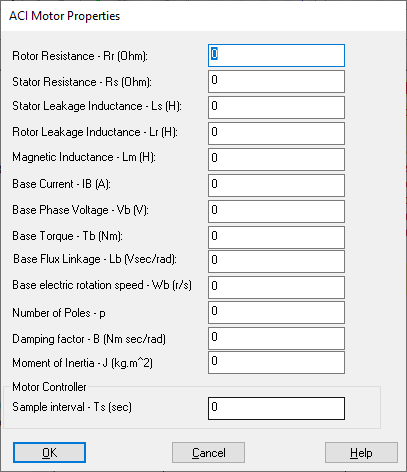## ACI Motor

Embedded Category: C2407, F280x

Block Category: TI 16-bit DMC, TI 32-bit DMC

Operating Mode Availability

Simulation mode: YES

C code generation mode: NO

The ACI Motor block implements a discrete equivalent of a 3-phase induction motor using trapezoidal approximation with predictor-corrector. The induction model is normalized by the adjustable base quantities of voltage, current, torque, frequency, and flux linkage. The induction motor is modeled in the stationary reference frame. The outputs of this module are the stator currents, rotor flux linkages, electromagnetic torque, electrically angular velocity, and actual rotor speed in rpm. These outputs are in per-unit except the actual rotor speed.Base Current: Indicates the maximum current flow at the rated load.

Base Electric Rotation Speed: Indicates the maximum target rotation.

Base Phase Voltage: Indicates the base phase voltage.

Base Torque: Indicates the base rated torque.

Damping Factor: Indicates the damping factor.

Magnetic Inductance: Indicates the magnetizing inductance of the load. Specify this value in henries.

Moment of Inertia: Indicates the inertia of the rotor.

Number of Poles: Indicates the number of poles in the motor.

Rotor Leakage Inductance: Indicates the rotor leakage inductance of the load. Specify this value in henries.

Rotor Resistance: Indicates the rotor resistance of the load. Specify this value in henries.

Sample Interval: Indicates the sampling interval of the motor controller.

Stator Leakage Inductance: Indicates the stator leakage.

Stator Resistance: Indicates the stator resistance.